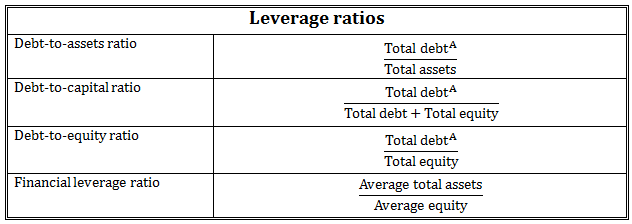# CFAI Reading 20 Question 3

Hey guys, really confused here. The question is about capitalizing leases and you have to calculate the new leverage ratio (D/E). The question gives you average assets= 2075 and average equity= 1156. The capitalized leases are worth 1,297. What is the new financial ratio? So what I did was add the capitalized leases to the average assets to get 3,372, I then subtracted the average equity to get liabilities of 2,216. I then computed D/E as 2,216/1,156 and arrived at 1.92.

The answer that CFAI gives you is “C is correct. The capitalized value of the leases is added to assets and liabilities but does not impact equity. On an adjusted basis, Silk Road’s financial leverage ratio = (2,075 + 1,297)/1,156 = 2.92” But doesn’t this just take assets/equity?

Precisely, financial leverage is one of the leverage ratios, and is interpreted as asset/equity (part of DuPont).